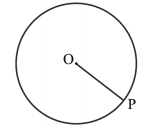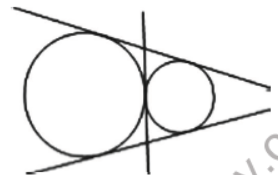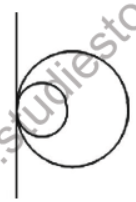# CBSE Class 10 Circles Important Formulas and concepts for exams

## Study Material for Class 10 Circles Chapter 10 Circles

Class 10 Circles students should refer to the following Pdf for Chapter 10 Circles in standard 10. These notes and test paper with questions and answers for Grade 10 Circles will be very useful for exams and help you to score good marks

### Class 10 Circles Chapter 10 Circles

CBSE Class 10 Circles Important Formulas and concepts for exams. There are many more useful educational material which the students can download in pdf format and use them for studies. Study material like concept maps, important and sure shot question banks, quick to learn flash cards, flow charts, mind maps, teacher notes, important formulas, past examinations question bank, important concepts taught by teachers. Students can download these useful educational material free and use them to get better marks in examinations.  Also refer to other worksheets for the same chapter and other subjects too. Use them for better understanding of the subjects.

Circle

The collection of all the points in a plane, which are at a fixed distance from a fixed point in the plane, is called a circle.

* The fixed point is called the centre of the circle and the fixed distance is called the radius of the circle. In the below figure, O is the centre and the length OP is the radius of the circle.* The line segment joining the centre and any point on the circle is also called a radius of the circle.

* A circle divides the plane on which it lies into three parts. They are: (i) inside the circle, which is also called the interior of the circle; (ii) the circle and (iii) outside the circle, which is also called the exterior of the circle. The circle and its interior make up the circular region.

* The chord is the line segment having its two end points lying on the circumference of the circle.

* The chord, which passes through the centre of the circle, is called a diameter of the circle.

* A diameter is the longest chord and all diameters have the same length, which is equal to two times the radius.

* A piece of a circle between two points is called an arc.

* The longer one is called the major arc PQ and the shorter one is called the minor arc PQ.

* The length of the complete circle is called its circumference.

* The region between a chord and either of its arcs is called a segment of the circular region or simply a segment of the circle. There are two types of segments also, which are the major segment and the minor segment.

* The region between an arc and the two radii, joining the centre to the end points of the arc is called a sector. The minor arc corresponds to the minor sector and the major arc corresponds to the major sector.

* In the below figure, the region OPQ is the minor sector and remaining part of the circular region is the major sector. When two arcs are equal, that is, each is a semicircle, then both segments and both sectors become the same and each is known as a semicircular region.Points to Remember :

A circle is a collection of all the points in a plane, which are equidistant from a fixed point in the plane.

Equal chords of a circle (or of congruent circles) subtend equal angles at the centre.

If the angles subtended by two chords of a circle (or of congruent circles) at the centre (corresponding centre) are equal, the chords are equal.

The perpendicular from the centre of a circle to a chord bisects the chord.

The line drawn through the centre of a circle to bisect a chord is perpendicular to the chord.

There is one and only one circle passing through three non-collinear points.

Equal chords of a circle (or of congruent circles) are equidistant from the centre (or corresponding centres).

Chords equidistant from the centre (or corresponding centres) of a circle (or of congruent circles) are equal.

If two arcs of a circle are congruent, then their corresponding chords are equal and conversely, if two chords of a circle are equal, then their corresponding arcs (minor, major) are congruent.

Congruent arcs of a circle subtend equal angles at the centre.

The angle subtended by an arc at the centre is double the angle subtended by it at any point on the remaining part of the circle

Angles in the same segment of a circle are equal.\

Angle in a semicircle is a right angle.

If a line segment joining two points subtends equal angles at two other points lying on the same side of the line containing the line segment, the four points lie on a circle.

The sum of either pair of opposite angles of a cyclic quadrilateral is 180°.

If the sum of a pair of opposite angles of a quadrilateral is 180°, then the quadrilateral is cyclic.

Secant to a Circle

A secant to a circle is a line that intersects the circle at exactly two points.

Tangent to a Circle

A tangent to a circle is a line that intersects the circle at only one point.

Given two circles, there are lines that are tangents to both of them at the same time.

If the circles are separate (do not intersect), there are four possible common tangents:If the two circles touch at just one point, there are three possible tangent lines that are common to both:If the two circles touch at just one point, with one inside the other, there is just one line that is a tangent to both:If the circles overlap - i.e. intersect at two points, there are two tangents that are common to both:If the circles lie one inside the other, there are no tangents that are common to both. A tangent to the inner circle would be a secant of the outer circle.The tangent to a circle is perpendicular to the radius through the point of contact.

The lengths of tangents drawn from an external point to a circle are equal.

The centre lies on the bisector of the angle between the two tangents.

*“If a line in the plane of a circle is perpendicular to the radius at its endpoint on the circle, then the line is tangent to the circle”.